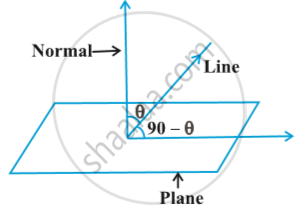# Angle Between Line and a Plane

## Definition

The angle between a line and a plane is the complement of the angle between the line and normal to the plane Fig.## Notes

Vector form  If the equation of the line is vec r = vec a + lambda vec b and the equation of the plane is vec r . vec n = d . Then the angle θ between the line and the normal to the plane is
cos θ = |(vec b . vec n)/(|vec b| . |vec n|)|
and so the angle φ between the line and the plane is given by 90 – θ, i.e.,
sin (90 – θ) = cos θ
i.e. sin φ = |(vec b . vec n)/(|vec b| . |vec n|)|  or

φ =sin ^ (–1)  |(bar b . bar n)/(|bar b| . |bar n|)|

If you would like to contribute notes or other learning material, please submit them using the button below.

### Shaalaa.com

3 Dimensional Geometry part 27 (Angle between line and plane) [00:06:14]
S
0%